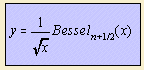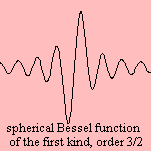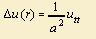# spherical Bessel function

## gamma

last updated: 2003-07-20The spherical Bessel function is found in the case of systems with spherical symmetry.
For instance in the situation of a three dimensional wave, which obeys the standard wave equation 1).

The function can easily expressed as a Bessel function, as we can see in the formula on top (omitting constants).

For the Bessel function of the first kind and the order (n) is equal to 0, the function is equivalent to the damped sine.

notes

1)